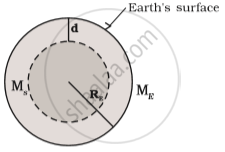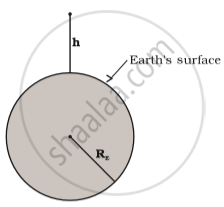# Acceleration Due to Gravity Below and Above the Surface of Earth

#### notes

Acceleration due to gravity below the surface of earth

• To calculate acceleration due to gravity below the surface of the earth (between the surface and centre of the earth).

Density of the earth is constant throughout. Therefore,

ρ = M/ (4/3π Re3)          ....(1)

where,

M= mass of the earth

Volume of sphere= 4/3π "R"_e^2

Re = radius of the earth.

As entire mass is concentrated at the centre of the earth.

Therefore density can be written as

rho = M_s(4/3π "R"_s^3)      ....(2)

Comparing equation (1) and (2)

"M"_e/"M"_s="R"_e^3/"R"_s^3      "where"  "R"_s= ("R"_e-"d")^3

d= distance of the body form the centre to the surface of the earth.

Therefore,

"M"_e/"M"_s="R"_e^3/("R"_e-"d")^3

"M"_s = "M"_e(("R"_e-"d")^3)/"R"_e^3        ...(3)

To calculate Gravitational force (F) between earth and point mass m at a depth d below the surface of the earth.Above figure shows the value of g at a depth d. In this case only the smaller sphere of radius ("R"_e- "d") contributes to g.

"F" ="GmM"_s/("R"_e-"d")^2

"g"="F"/"m"  where g=acceleration due to gravity at point 'd' below the surface of the earth.

"g"="GM"_s/("R"_e-"d")^2

Putting the value of "M"_s from Eq.(3)

="GM"_e("R"_e-"d")^3/("R"_e^3("R"_e-"d")^2)

="GM"_e(R_e-d)/R_e^3

W.k.t.    "g"="GM"_e/"R"_e^2

g(d)="GM"_e/R_e^3("R"_e-"d")

=g((R_e-d)/R_e)=g(1-d/R_e)

Acceleration due to gravity above the surface of the earth:

• To calculate the value of acceleration due to gravity of a point mass m at a height h above the surface of the earth.

• Force of gravitation between the object and the earth will beF(h)=("GM"_Em)/(R_E + h)^2

The acceleration experienced by the point mass is ("F"("h"))/"m"≡"g"("h")

"g"(h)=("F"(h))/m="GM"_E/("R"_E + h)^2 ...(1)

This is cleary less than the value of g on the surface of earth: "g"="GM"_E/"R"_E^2 for h<<RE, we can expand the RHS of Eq.(1)

g(h)="GM"_E/(R_E^2 (1+h/R_E)^2)

=g(1+h/"R"_E)^-2

For "h"/"R"_E<<1. Using binomial expression,

"g"(h)cong"g"(1-(2h)/R_E)

Conclusion: The value of acceleration due to gravity varies on the surface, above surface and below the surface of the earth.

If you would like to contribute notes or other learning material, please submit them using the button below.